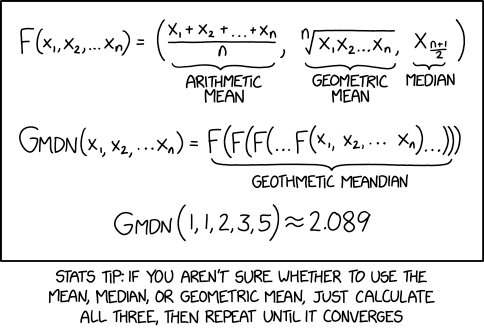## information loss from the median

Posted in Books, Kids, Statistics with tags , , , , , , on April 19, 2022 by xi'anAn interesting side item from a X validated question about calculating the Fisher information for the Normal median (as an estimator of the mean). While this information is not available in closed form, it has a “nice” expression$1+n\mathbb E[Z_{n/2:n}\varphi(Z_{n/2:n})]-n\mathbb E[Z_{n/2:n-1}\varphi(Z_{n/2:n-1})]+$$\frac{n(n-1)}{n/2-2}\varphi(Z_{n/2-2:n-2})^2+\frac{n(n-1)}{n-n/2-1}\varphi(Z_{n/2:n-2})^2$

which can easily be approximated by simulation (much faster than by estimating the variance of said median). This shows that the median is about 1.57 less informative than the empirical mean. Bonus points for computing the information brought by the MAD statistic! (The information loss against the MLE is 2.69,  since the Monte Carlo ratio of their variances is 0.37.)

## analogy between mean and median [X validated]

Posted in Books, Kids, Statistics with tags , , , on February 6, 2022 by xi'an

A recent question on X validated was looking rather naïvely for the median being equal to the mean, as earlier questions there, but this made me realise a certain symmetry in the two notions, namely that the median ς is solution of the balance condition$\int_{-\infty}^\varsigma f(d)\text{d}x = \int^{+\infty}_\varsigma f(xd)\text{d}x$

namely the areas under the density are equal, while the mean μ is solution of a parallel balance condition$\int_{-\infty}^\mu F(d)\text{d}x = \int^{+\infty}_\mu (1-F)(x)\text{d}x$

which is an equality of the same kind, i.e., of areas under the cdf or its complement…. Courtesy of the general representation$\mathbb E[X] = \int_0^{\infty} (1-F)(x) \text dx - \int_{-\infty}^0 F(x) \text dx$

The general problem of having the mean and median equal is not particularly interesting as it is a matter of parameterisation. For instance, using the cdf transform turns the random variate X into a Uniform F(X), with mean and median equal to ½.

## meandering

Posted in Books, Kids, R, Statistics with tags , , , , , , , on March 12, 2021 by xi'anA bit of a misunderstanding from Randall Munroe and then some: the function F returns a triplet, hence G should return a triplet as well. Even if the limit does return three identical values. And he should have also included the (infamous) harmonic mean! And the subtext (behind the picture) mentions random forest statistics, using every mean one can think of and dropping those that are doing worse, while here all solutions return the same value, hence do not directly discriminate between the averages (and there is no objective function to create the nodes in the trees, &tc.).

Here is a test R code including the harmonic mean:

xkcd=function(x)c(mean(x),exp(mean(log(x))),median(x),1/mean(1/x))
xxxkcd=function(x,N=10)ifelse(rep(N==1,4),xkcd(x),xxxkcd(xkcd(x),N-1))
xxxkcd(rexp(11))
 1.018197 1.018197 1.018197 1.018197


## double if not exponential

Posted in Books, Kids, Statistics, University life with tags , , , , , , on December 10, 2020 by xi'anIn one of my last quizzes for the year, as the course is about to finish, I asked whether mean or median was the MLE for a double exponential sample of odd size, without checking for the derivation of the result, as I was under the impression it was a straightforward result. Despite being outside exponential families. As my students found it impossible to solve within the allocated 5 minutes, I had a look, could not find an immediate argument (!), and used instead this nice American Statistician note by Robert Norton based on the derivative being the number of observations smaller than θ minus the number of observations larger than θ.  This leads to the result as well as the useful counter-example of a range of MLE solutions when the number of observations is even.

## sampling the mean

Posted in Kids, R, Statistics with tags , , , , , on December 12, 2019 by xi'an

A challenge found on the board of the coffee room at CEREMADE, Université Paris Dauphine:

When sampling with replacement three numbers in {0,1,…,N}, what is the probability that their average is (at least) one of the three?

With a (code-golfed!) brute force solution of

mean(!apply((a<-matrix(sample(0:n,3e6,rep=T),3)),2,mean)-apply(a,2,median))


producing a graph pretty close to 3N/2(N+1)² (which coincides with a back-of-the-envelope computation):